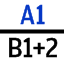## Render using Cell References

Renders the selected cell by using the absolute cell references. Therefore, if a formula uses a Name that refers to a cell or range, then it is converted back into the 'A1' reference style.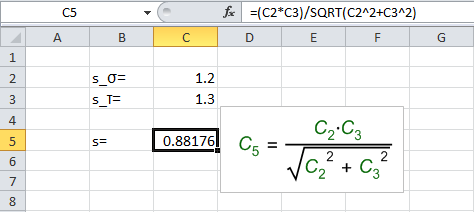Example 1: Style: Excel Buttons:

### Appearance

• Variables that refer to the cell using the 'A1' style coordinate system (i.e. column letter followed by row number) are shown in Green text by default (all color defaults can be changed in settings).
• Named Excel cells, which can be used to refer to specific cells within a formulas, are replaced with the 'A1' style cell reference in the rendered equation - useful for finding the exact cells that are being referenced.

• Commond to buttons:
• Function names, e.g. sin() or min(), are shown in Dark Red text by default.
• Constant values, operators and other symbols are shown in Black by default.

### Usage

New Equations

With new rendered equations, the system will use the current defaults. These include all the formatting pane options and the current state of the following buttons:

• Display Assignment (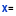)
• Display Units (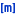)
• Box (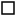)

Existing Equations:

Where there is an existing rendered equation for a formula, the default action is to reuse as many of the properties from the previous equation as possible, including the:

• Display Assignment ()
• Display Units ()
• Box ()
• Format (i.e. gif, emf or text)
• Position of the equation. Note: Rendered equations can be dragged to any other location on the same sheet and also resized.
• The Font and Font size (i.e. Arial, Times New Roman etc)
• The Style (i.e. Excel, Math, Matrix)

Shift Key: Press the shift-key when rendering a formula to ensure the newly rendered equation uses the default settings shown on the toolbar.

Ctrl Key: Press the ctrl-key at the same time as selecting the Cell Reference to toggle this button on/off. When permanently enabled, newly rendered equations will automatically include this rendering option alongside any other form of the equation that the user selects.

Updating: All features of any rendered equation can be changed, by selecting one or more rendered equations (or formula cell) and clicking the alternative formatting required from the toolbar.

Note: These Usage instruction apply equally to all the main Rendering buttons.

### Example 1

Download the 'Example spreadsheet' and follow these steps:

• Select tab 'Example 1'
• Select cell C5, which contains the formula "=(C2*C3)/SQRT(C2^2+C3^2)".
• Click the Cell Reference (Ref) button in the Render section.
• The rendered equation will appear in the first empty space to the right of the selected cell.Example 1: Style: Excel Buttons: